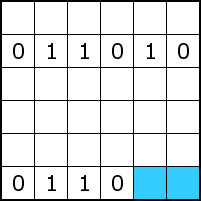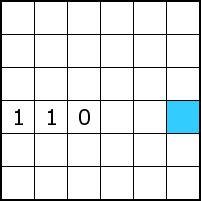# Tips

Find pairs

Because at most two equal numbers immediately next to or below each other are allowed, pairs can be supplemented with the other number. Thus, in the example below, both blue boxes should contain a zero.Avoid trios

If two cells contain the same number with an empty cell in between, this empty cell should contain the other number. The blue cell in the example below should contain a one, because otherwise a trio appears.Complete rows and columns

Each row and each column should contain an equal number of zeros and ones. If the required number of zeros is reached in a row or a column, the remaining cells should contain a one, and vice versa. In the example below, each row and each column should contain 3 ones and 3 zeros. This implies that the blue cell in the third row should be a one, and that both blue cells in the third column should be zeros.Eliminate impossible combinations based on completed rows and columns

In the example below, there are a one and a zero missing in the bottom row. If the one is filled in in the left blue cell and the zero in the right blue cell, the bottom row becomes equal to the second row. Because this is not allowed, the left blue cell should be a zero, and the right blue cell a one.Eliminate other impossible combinations

In the situation below there is only a single one missing in the fourth row. If this one is placed in the blue cell, all cells in between should be zeros, resulting in a trio (three zeros in a row). Because this is not allowed, the blue cell should contain a zero.BinaryPuzzle.com uses cookies to provide you with an optimal user experience.